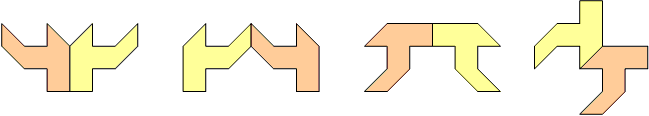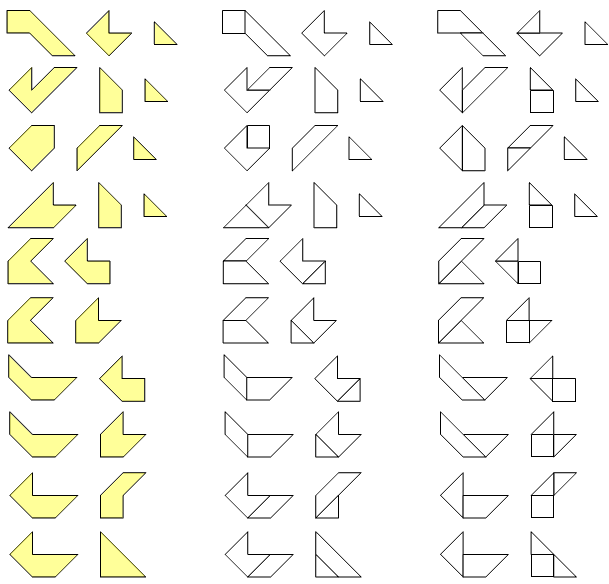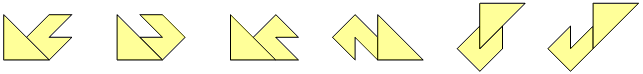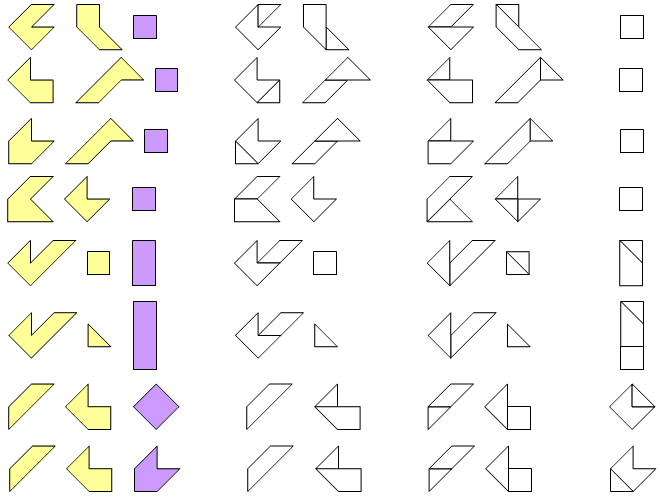Symmetric patterns for Pygram
by Helmut Postl

I think, there are too many to list all of them Here I will show some general constructions how to build symmetric patterns.

Double subpatterns
We want to construct a set of small patterns which can be filled exactly twice by all pygram pieces. For example:These three subpatterns can be put together in any way (there are lots!) to make a “half-pattern”, for example:One of the two copies of this half-pattern is flipped over, and then the two half-patterns can be arranged in several ways to make a symmetric whole pattern (here the first example is used):Here are more examples for double subpatterns:Double subpatterns with symmetric subpattern
Another way to construct symmetric patterns is to use a small symmetric subpattern which comes to lie on the axis of symmetry. The double subpatterns are arranged symmetrically as above. For example:Again, the two subpatterns can be put together in any way to make a “half-pattern”, for example:Again, one of the two copies of this half-pattern is flipped over, and then the two half-patterns can be arranged in several ways with the symmetric subpattern to make a symmetric whole pattern (again, the first example is used):Here are more examples for double subpatterns with symmetric subpattern:Wat is het toch plezant om slimme vrienden te hebben. Bedankt Helmut!